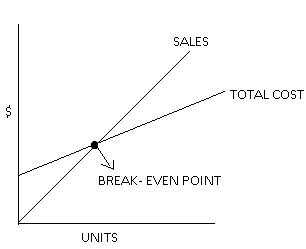# Break Even Point

## Break-Even Point Definition

The Break-even point is the level of production where the company’s total revenues and expenses are equal. At the BEP, the revenue of the company by the sale of manufactured products is equal to the total costs incurred in manufacturing the product. In accounting terms, at this point, the company’s total profit is zero. So it is a situation where there is no profit, no loss to the company.

Take an example for BEP; if the total revenues of ABC Ltd. are \$ 7,000 and total expenses also equal to \$ 7,000, we can say that ABC Ltd. is working at the BEP.

## Formula

1. The formula to calculate the break-even point in terms of the number of units is:

Break-Even Point in Units = Fixed Cost / Sales Price Per Unit – Variable Cost Per Unit

There is another simplified formula for calculating the BEP in terms of the number of units. That is,

Break-Even Point in Units = Fixed Costs / Contribution Margin Per Unit

Where,

Contribution Margin Per Unit = Sales Price Per Unit – Variable Cost Per Unit

Let us understand the meaning of contribution margin in brief. Contribution margin means revenue minus variable expenses. With contribution margin, you come to know how much of the company’s revenue will be available to pay for the fixed expenses and to generate the net income.

1. The formula for calculating BEP in terms of money is:

Break-Even Point = Break-Even Point in Units x Sales Price Per Unit

## ExampleCalculate the BEP in terms of dollars and sales units from the information given below:

Fixed Cost: \$ 10,000

Price Per Unit: \$ 20

Variable Cost Per Unit: \$ 10

Solution

Let us put the given information in the formula for calculating the BEP in terms of sales units:

Break-Even Point in Units = Fixed Costs / Sales Price Per Unit – Variable Cost Per Unit

= \$ 10,000 / \$ 20 – \$ 10

= 1,000 units

Break Even Point in Terms of Money = Break Even Point in Units x Sales Price Per Unit

= 1,000 x \$ 20

= \$ 20,000

## Uses

A Break-even point is useful in a lot of situations like:

• It helps determine the impact on profit if physical labor (variable cost) is substituted by automation (fixed cost).
• It helps to determine the effect of the change in the price of a product on the profits.
• If the business suffers a sales downturn at any point, BEP determines the number of losses that a business could sustain.
• It helps to determine the maximum profit that can be earned with the remaining capacity left after reaching the BEP.

## Conclusion

Every business functions to earn profits. If it is unable to earn a profit, it aims to break even. BEP is a point where revenues and expenses of a business are equal, and it is the next best position for a company that is not earning profits. It is the minimum point below which the company will start incurring losses, so the company’s management strives toward working above the BEP.

Quiz on Break-Even point.

Let’s review what you read here with a quick quiz test.Sanjay Borad is the founder & CEO of eFinanceManagement. He is passionate about keeping and making things simple and easy. Running this blog since 2009 and trying to explain "Financial Management Concepts in Layman's Terms".

### 2 thoughts on “Break Even Point”

1.Concept clearly explianed. Nice??

2.